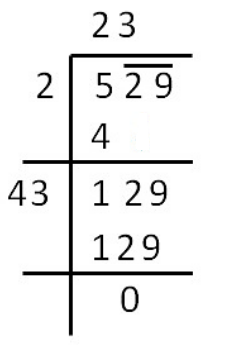Find the square root of the following number by Division method: 529Verified
148.8k+ views
Hint: Here, use the division method by grouping 529 as 5 29 and do the process of division. At the last to check whether the result obtained is correct or not, multiply the result by itself to get 529.

Here, we have divided the solution into steps.
Step I: Write the given number 529 in two parts as 5 29 by grouping the numbers in pairs, always starting from the right end of the given number. (Here, start from the number 5 ).
Step II: Find the greatest positive number whose square is less than (or equal to) 5. Here it is 2, as ${2^2} < 5$.
Step III: Write 2 in place of the quotient (or on the top), and also write 2 as a divisor.
Step IV: Multiply 2 on the top with the divisor 2 and write the result i.e. 4 under 5 and subtract as we do in the division. The remainder obtained is 1.
Step V: Write down the pair 29 by the side of the remainder 1, we get 129.
Step VI: Double 2 and take the resulting number 4. Find that number y such that 4y × y is just less than or equal to 129.
Here, we have 42×2=84 and 43×3=129 and so y = 3.
Step VII: Now, we have 43 as the next divisor and put 3 on the top along with 2. Write the product 43 × 3=129 under 129 and subtract again as we do in division. Since the remainder is 0, the division is complete. Hence, $\sqrt {529}$ =23
See below:Also, 23 × 23 = 529, hence our answer is correct.

Note:
The division method is useful for finding square roots of large numbers as the factorization method is lengthy for big numbers.